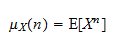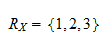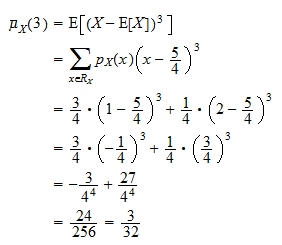StatLect

# Moments of a random variable

This lecture introduces the notion of moment of a random variable.## Moment

The-th moment of a random variable is the expected value of its-th power.

Definition Letbe a random variable. Let. If the expected valueexists and is finite, thenis said to possess a finite-th moment andis called the-th moment of. Ifis not well-defined, then we say thatdoes not possess the-th moment.

The following example shows how to compute a moment of a discrete random variable.

Example Letbe a discrete random variable having supportand probability mass functionThe third moment ofcan be computed as follows:## Central moment

The-th central moment of a random variableis the expected value of the-th power of the deviation offrom its expected value.

Definition Letbe a random variable. Let. If the expected valueexists and is finite, thenis said to possess a finite-th central moment andis called the-th central moment of.

The next example shows how to compute the central moment of a discrete random variable.

Example Letbe a discrete random variable having supportand probability mass functionThe expected value ofisThe third central moment ofcan be computed as follows:## More details

The following subsections contain more details about moments.

### Multivariate generalization

A generalization of the concept of moment to random vectors is introduced in the lecture entitled Cross-moments.

### Computation

The moments of a random variable can be easily computed by using either its moment generating function, if it exists, or its characteristic function (see the lectures entitled Moment generating function and Characteristic function).